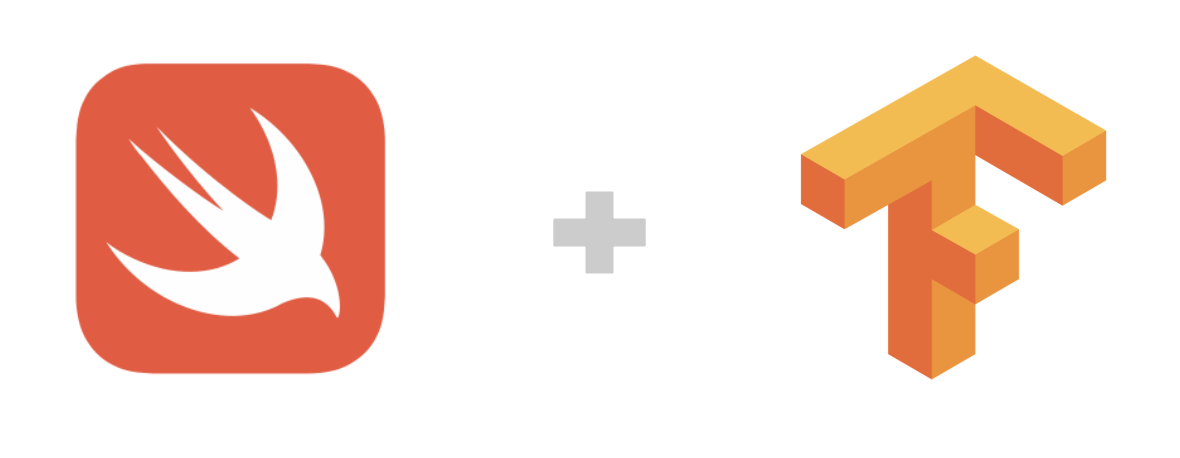# Swift for TensorFlow (S4TF) (Huan）¶「Swift for TensorFlow is an attempt to change the default tools used by the entire machine learning and data science ecosystem.」

– Jameson Toole, Co-founder & CTO of Fritz.ai

## S4TF 簡介¶

Google 推出的 Swift for TensorFlow （簡稱 S4TF）是專門針對 TensorFlow 優化過的 Swift 版本。（目前處在 Pre-Alpha 階段）

Swift 語言創始人 Chris Lattner

Swift 語言是 Chris Lattner 在蘋果公司工作時創建的。 現在 Chris Lattner 在 Google Brain 工作，專門從事深度學習的研究，並為 Swift 重寫了編譯器，為 TensorFlow 做定製優化。

### 為什麼要使用 Swift 進行 TensorFlow 開發¶

1. 開發效率高：強類型語言，能夠靜態檢查變數類型

2. 遷移成本低：與 Python，C，C++ 能夠無縫結合

3. 執行性能高：能夠直接編譯為底層硬體程式碼

4. 專門為機器學習打造：語言原生支持自動微分系統

## S4TF 環境配置¶

### 在 Colaboratory 中快速體驗 Swift for TensorFlow¶

Google 的 Colaboratory 可以直接支援 Swift 語言的執行環境。可以 點此 直接打開一個空白的，具備 Swift 執行環境的 Colab Notebook ，這是立即體驗 Swift for TensorFlow 的最方便的辦法。

### 在 Docker 中快速體驗 Swift for TensorFlow¶

1. 開啟一個 S4TS 的 Jupyter Notebook

在終端機中執行 `nvidia-docker run -ti --rm -p 8888:8888 --cap-add SYS_PTRACE -v "\$(pwd)":/notebooks zixia/swift` 來啟動 Jupyter ，然後根據提示的 URL ，打開瀏覽器執行即可。

2. 執行一個本機的 Swift 程式碼文件

為了執行本機的 `s4tf.swift` 文件，我們可以用如下 docker 指令：

```nvidia-docker run -ti --rm --privileged --userns=host \
-v "\$(pwd)":/notebooks \
zixia/swift \
swift ./s4tf.swift
```

## S4TF 基礎使用¶

Swift 是動態強型態語言，也就是說 Swift 支持通過編譯器自動檢測類型，同時要求變數的使用要嚴格符合定義，並且變數都必須先定義後才能使用。

```var n = 42
n = "string"
```

```Cannot assign value of type 'String' to type 'Int'
```

```import TensorFlow

// 宣告兩個Tensor
let x = Tensor<Float>()
let y = Tensor<Float>()

// 對兩個 Tensor 做加法運算
let w = x + y

// 輸出結果
print(w)
```

`Tensor<Float>` 中的 `<Float>`

### 在 Swift 中使用標準的 TensorFlow API¶

`import TensorFlow` 之後，就可以在 Swift 語言中，使用核心的 TensorFlow API。

1. 處理數字和矩陣的程式碼，API 與 TensorFlow 高度保持了一致：

```let x = Tensor<BFloat16>(zeros: [32, 128])
let h1 = sigmoid(matmul(x, w1) + b1)
let h2 = tanh(matmul(h1, w1) + b1)
let h3 = softmax(matmul(h2, w1) + b1)
```
1. 處理 Dataset 的程式碼，基本上，將 Python API 中的 `tf.data.Dataset` 同名函數直接改寫為 Swift 語法即可直接使用：

```let imageBatch = Dataset(elements: images)
let labelBatch = Dataset(elements: labels)
let zipped = zip(imageBatch, labelBatch).batched(8)

let imageBatch = Dataset(elements: images)
let labelBatch = Dataset(elements: labels)
for (image, label) in zip(imageBatch, labelBatch) {
let y = matmul(image, w) + b
let loss = (y - label).squared().mean()
print(loss)
}
```

`matmul()` 的別名： `•`

### 在 Swift 中直接載入 Python 語言函式庫¶

Swift 語言支持直接載入 Python 函數函式庫（比如 NumPy），也支持直接載入系統動態鏈接函式庫，很方便的做到即導入使用。

import Python

let np = Python.import(“numpy”) let x = np.array([[1, 2], [3, 4]]) let y = np.array([11, 12]) print(x.dot(y))

```[35 81]
```

```import Glibc
let x = malloc(18)
memcpy(x, "memcpy from Glibc", 18)
free(x)
```

### 語言原生支持自動微分¶

```@differentiable
func frac(x: Double) -> Double {
return 1/x
}

```

```-4.0
```

Swift 函數宣告中的參數名稱和類型

Swift 使用 `func` 宣告一個函數。在函數的參數中，變數名的冒號後面代表的是 “參數類型”；在函數的參數和函數體（`{}`） 之前，還可以通過箭頭（`->`）來指定函數 `返回值的類型`

### MNIST數字分類¶

1. 首先，導入 S4TF 模組 `TensorFlow`、Python 橋接模組 `Python`，基礎模組 `Foundation` 和 MNIST 資料集模組 `MNIST`

```import TensorFlow
import Python
import Foundation

import MNIST
```

Swift MNIST Dataset 模組

Swift MNIST Dataset 模組是一個簡單易用的 MNIST 資料集載入模組，基於 Swift 語言，提供了完整的資料集載入 API。項目 Github：https://github.com/huan/swift-MNIST

1. 宣告一個最簡單的 MLP 神經網路架構，將輸入的 784 個圖像資料，轉換為 10 個神經元的輸出：

```struct MLP: Layer {
// 定義模型的輸入、輸出資料類型
typealias Input = Tensor<Float>
typealias Output = Tensor<Float>

// 定義 flatten 層，將二維矩陣展開為一堆陣列
var flatten = Flatten<Float>()
// 定義全連接層，輸入為 784 個神經元，輸出為 10 個神經元
var dense = Dense<Float>(inputSize: 784, outputSize: 10)

@differentiable
public func callAsFunction(_ input: Input) -> Output {
var x = input
x = flatten(x)
x = dense(x)
return x
}
}
```

Swift 參數標籤

Swift 中，每個函數參數都有一個 參數標籤 (Argument Label) 以及一個 參數名稱 (Parameter Name)。 參數標籤 主要應用在調用函數的情況，使得函數的實參與真實命名相關聯，更加容易理解實際參數的意義。同時因為有 參數標籤 的存在，確切的順序是可以隨意改變的。

1. 接下來，我們實例化這個 MLP 神經網路模型，實例化 MNIST 資料集，並將其存入 `imageBatch``labelBatch` 變量：

```var model = MLP()
let optimizer = Adam(for: model)

let mnist = MNIST()
let ((trainImages, trainLabels), (testImages, testLabels)) = mnist.loadData()

let imageBatch = Dataset(elements: trainImages).batched(32)
let labelBatch = Dataset(elements: trainLabels).batched(32)
```
1. 然後，我們通過對資料集的循環，計算模型的梯度 `grads` 並通過 `optimizer.update()` 來反向傳播更新模型的參數，進行訓練：

```for (X, y) in zip(imageBatch, labelBatch) {
// 計算梯度
let grads = gradient(at: model) { model -> Tensor<Float> in
let logits = model(X)
return softmaxCrossEntropy(logits: logits, labels: y)
}

// 優化器根據梯度更新模型參數
}
```

Swift 閉包函數（Closure）

Swift 的閉包函數宣告為：`{ (parameters) -> return type in statements }`，其中：`parameters` 為閉包接受的參數，`return type` 為閉包運行完畢的返回值類型，`statements` 為閉包內的運行程式碼。

Swift 尾隨閉包語法 (Trailing Closure Syntax)

Swift 輸入輸出參數 (In-Out Parameters)

1. 最後，我們使用訓練好的模型，在測試資料集上進行檢查，得到模型的準確度：

```let logits = model(testImages)
let acc = mnist.getAccuracy(y: testLabels, logits: logits)

print("Test Accuracy: \(acc)" )
```

```Downloading train-images-idx3-ubyte ...